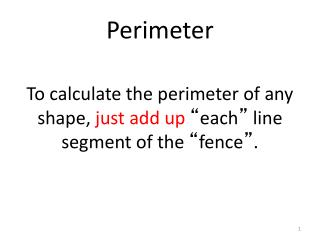DownloadDownload PresentationPerimeter

# Perimeter

Télécharger la présentation## Perimeter

- - - - - - - - - - - - - - - - - - - - - - - - - - - E N D - - - - - - - - - - - - - - - - - - - - - - - - - - -
##### Presentation Transcript

1. Perimeter To calculate the perimeter of any shape, just add up “each” line segment of the “fence”.

2. Perimeter • Triangles have 3 sides…add up each sides length. 8 8 8+8+8=24 The Perimeter is 24. 8

3. Perimeter A square has 4 sides for the fence. 12 12+12+12+12=48 The perimeter is 48. 12 12 12

4. Area • Area is the ENTIRE INSIDE of a shape • It is always measured in “squares” (sq. inch, sqft)

5. AREA • Square units means“that” many squares fit inside that shape (if measured in feet…it’s feet…if meters…it’s meters. In this example the area is 4 square units…note 4 squares fit) 1 2 3 4